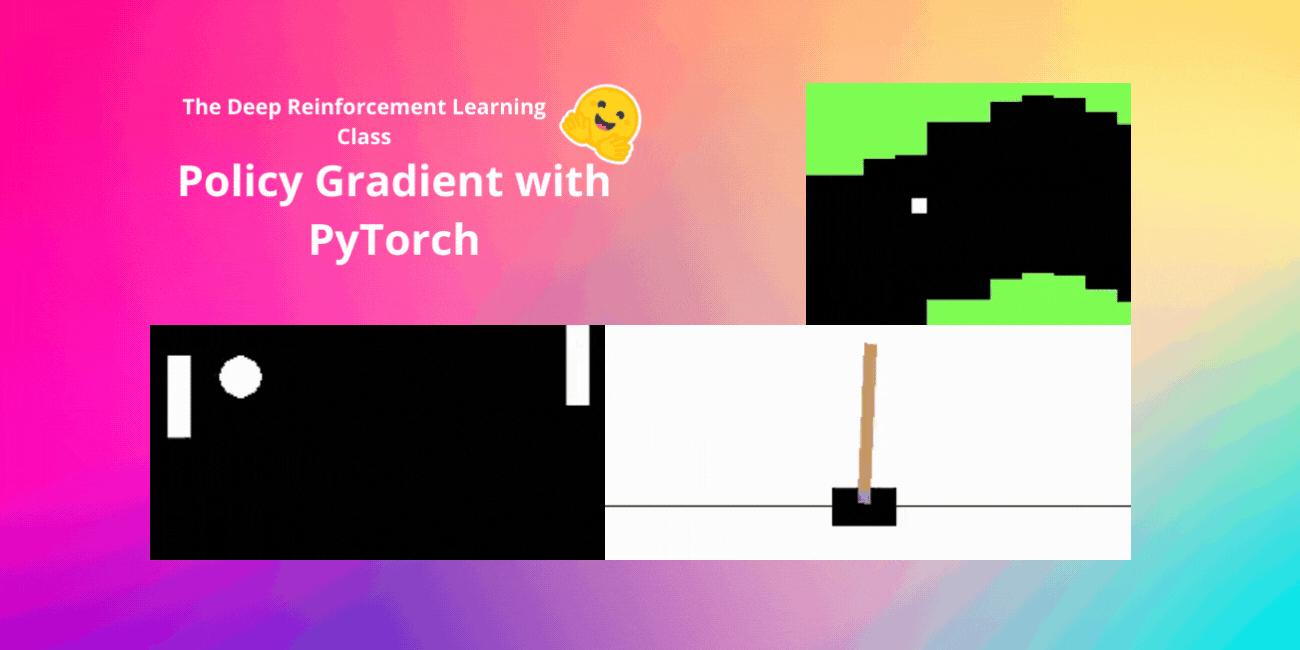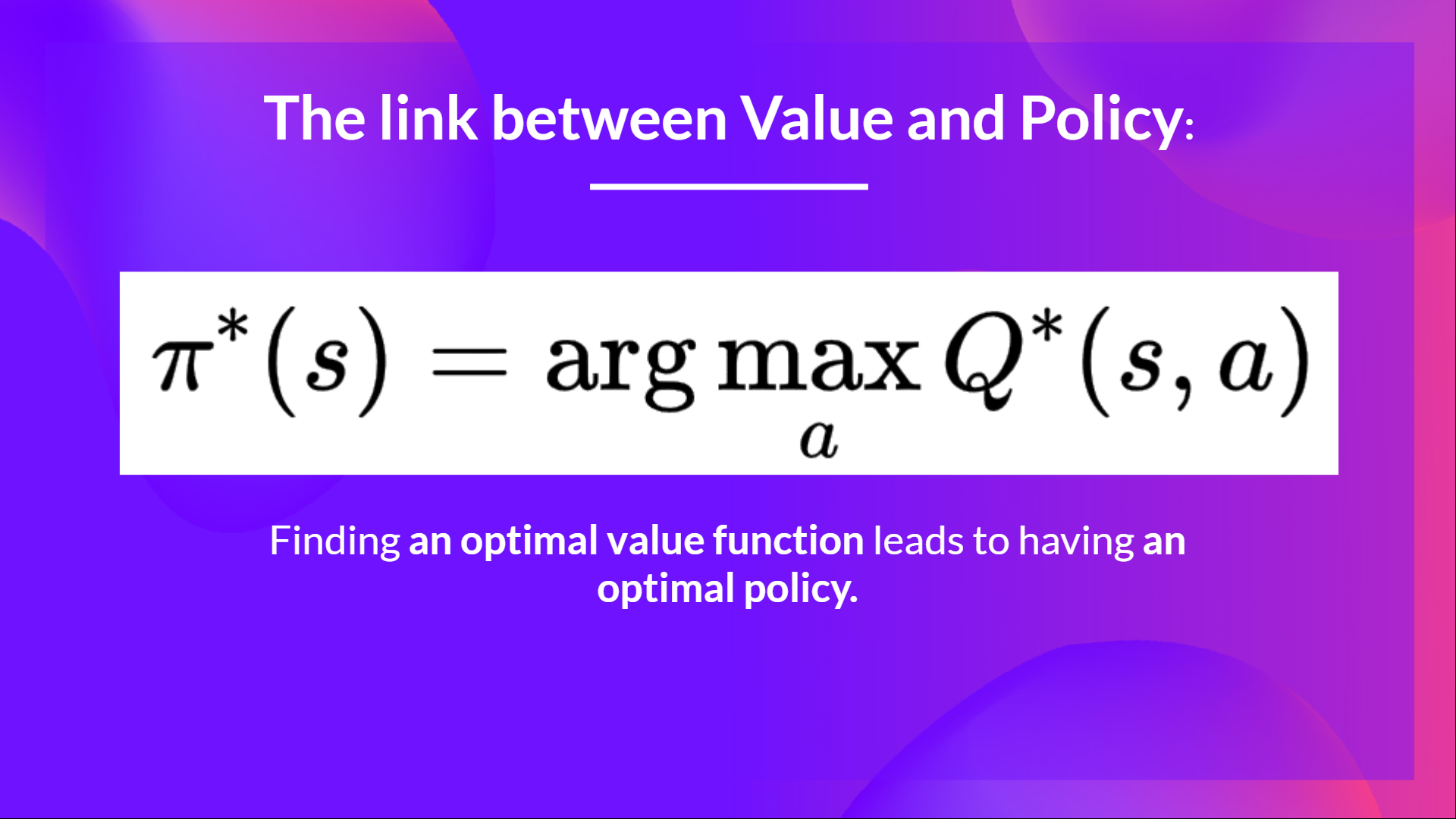## Unit 5, of the Deep Reinforcement Learning Class with Hugging Face 🤗

This article is part of the Deep Reinforcement Learning Class. A free course from beginner to expert. Check the syllabus here.In the last unit, we learned about Deep Q-Learning. In this value-based Deep Reinforcement Learning algorithm, we used a deep neural network to approximate the different Q-values for each possible action at a state.

Indeed, since the beginning of the course, we only studied value-based methods, where we estimate a value function as an intermediate step towards finding an optimal policy.Because, in value-based, π exists only because of the action value estimates, since policy is just a function (for instance, greedy-policy) that will select the action with the highest value given a state.

But, with policy-based methods, we want to optimize the policy directly without having an intermediate step of learning a value function.

So today, we'll study our first Policy-Based method: Reinforce. And we'll implement it from scratch using PyTorch. Before testing its robustness using CartPole-v1, PixelCopter, and Pong.

Let's get started,

Policy-Gradient is a subclass of Policy-Based Methods, a category of algorithms that aims to optimize the policy directly without using a value function using different techniques. The difference with Policy-Based Methods is that Policy-Gradient methods are a series of algorithms that aim to optimize the policy directly by estimating the weights of the optimal policy using Gradient Ascent.

### An Overview of Policy Gradients

Why do we optimize the policy directly by estimating the weights of an optimal policy using Gradient Ascent in Policy Gradients Methods?

Remember that reinforcement learning aims to find an optimal behavior strategy (policy) to maximize its expected cumulative reward.

We also need to remember that a policy is a function that given a state, outputs, a distribution over actions (in our case using a stochastic policy).

Our goal with Policy-Gradients is to control the probability distribution of actions by tuning the policy such that good actions (that maximize the return) are sampled more frequently in the future.

Let’s take a simple example:

• We collect an episode by letting our policy interact with its environment.

• We then look at the sum of rewards of the episode (expected return). If this sum is positive, we consider that the actions taken during the episodes were good: Therefore, we want to increase the P(a|s) (probability of taking that action at that state) for each state-action pair.

The Policy Gradient algorithm (simplified) looks like this:

But Deep Q-Learning is excellent! Why use policy gradient methods?

There are multiple advantages over Deep Q-Learning methods. Let's see some of them:

1. The simplicity of the integration: we can estimate the policy directly without storing additional data (action values).

2. Policy gradient methods can learn a stochastic policy while value functions can't.

This has two consequences:

a. We don't need to implement an exploration/exploitation trade-off by hand. Since we output a probability distribution over actions, the agent explores the state space without always taking the same trajectory.

b. We also get rid of the problem of perceptual aliasing. Perceptual aliasing is when two states seem (or are) the same but need different actions.

Let's take an example: we have an intelligent vacuum cleaner whose goal is to suck the dust and avoid killing the hamsters.

Our vacuum cleaner can only perceive where the walls are.

The problem is that the two red cases are aliased states because the agent perceives an upper and lower wall for each.

Under a deterministic policy, the policy will either move right when in a red state or move left. Either case will cause our agent to get stuck and never suck the dust.

Under a value-based RL algorithm, we learn a quasi-deterministic policy ("greedy epsilon strategy"). Consequently, our agent can spend a lot of time before finding the dust.

On the other hand, an optimal stochastic policy will randomly move left or right in grey states. Consequently, it will not be stuck and will reach the goal state with a high probability.

1. Policy gradients are more effective in high-dimensional action spaces and continuous actions spaces

Indeed, the problem with Deep Q-learning is that their predictions assign a score (maximum expected future reward) for each possible action, at each time step, given the current state.

But what if we have an infinite possibility of actions?

For instance, with a self-driving car, at each state, you can have a (near) infinite choice of actions (turning the wheel at 15°, 17.2°, 19,4°, honking, etc.). We'll need to output a Q-value for each possible action! And taking the max action of a continuous output is an optimization problem itself!

Instead, with a policy gradient, we output a probability distribution over actions.

• Policy gradients converge a lot of time on a local maximum instead of a global optimum.
• Policy gradient goes faster, step by step: it can take longer to train (inefficient).
• Policy gradient can have high variance (solution baseline).

👉 If you want to go deeper on the why the advantages and disadvantages of Policy Gradients methods, you can check this video.

Now that we have seen the big picture of Policy-Gradient and its advantages and disadvantages, let's study and implement one of them: Reinforce.

## Reinforce (Monte Carlo Policy Gradient)

Reinforce, also called Monte-Carlo Policy Gradient, uses an estimated return from an entire episode to update the policy parameter $\theta$.

We have our policy π which has a parameter θ. This π, given a state, outputs a probability distribution of actions.

Where $\pi_\theta(a_t|s_t)$ is the probability of the agent selecting action at from state st, given our policy.

But how do we know if our policy is good? We need to have a way to measure it. To know that we define a score/objective function called $J(\theta)$.

The score function J is the expected return:

Remember that policy gradient can be seen as an optimization problem. So we must find the best parameters (θ) to maximize the score function, J(θ).

To do that we’re going to use the Policy Gradient Theorem. I’m not going to dive on the mathematical details but if you’re interested check this video

The Reinforce algorithm works like this: Loop:

• Use the policy $\pi_\theta$ to collect an episode $\tau$
• Use the episode to estimate the gradient $\hat{g} = \nabla_\theta J(\theta)$
• Update the weights of the policy: $\theta \leftarrow \theta + \alpha \hat{g}$

The interpretation we can make is this one:

• $\nabla_\theta log \pi_\theta(a_t|s_t)$ is the direction of steepest increase of the (log) probability of selecting action at from state st. => This tells use how we should change the weights of policy if we want to increase/decrease the log probability of selecting action at at state st.
• $R(\tau)$: is the scoring function:
• If the return is high, it will push up the probabilities of the (state, action) combinations.
• Else, if the return is low, it will push down the probabilities of the (state, action) combinations.

Now that we studied the theory behind Reinforce, you’re ready to code your Reinforce agent with PyTorch. And you'll test its robustness using CartPole-v1, PixelCopter, and Pong.

Start the tutorial here 👉 https://colab.research.google.com/github/huggingface/deep-rl-class/blob/main/unit5/unit5.ipynb

---

Congrats on finishing this chapter! There was a lot of information. And congrats on finishing the tutorial. You’ve just coded your first Deep Reinforcement Learning agent from scratch using PyTorch and shared it on the Hub 🥳.

It's normal if you still feel confused with all these elements. This was the same for me and for all people who studied RL.

Take time to really grasp the material before continuing.

Don't hesitate to train your agent in other environments. The best way to learn is to try things on your own!